Default constructors

Default constructors help you to quickly instanciate the objects you would like to do math with. An example: Τ★ creates tensors of arbitrary rank.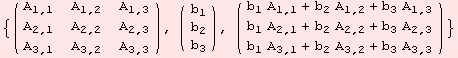As we demonstrate above, Τ★ is used to obtain general expression for formulas in linear algebra. Functions of the DGkernel acting as default constructors, usually have a 5-star ★ attached in the command name. Τ★ instanciates tensors of arbitrary tensor rank.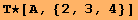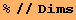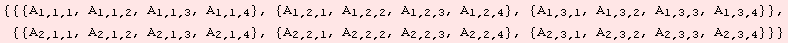To set up an symmetric (0,2)-tensor with symbolic entries, we use ΤS. For skew-symmetric matrices, please use ΤA★.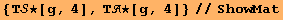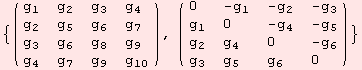The Riemannian curvature (1,3)-tensor field R of a semi-Riemannian manifold becomes a (1,3)-tensor when restricted to the tangent space of a point x. The tensor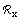has special symmetries. Some of them are allready encoded in the command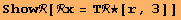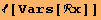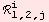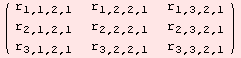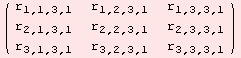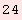Instead of 3·3·3=27 variable, R comes with 24. If we input the scalar-product, which originates from the metric at the point x, we get the exact number of degrees of freedom, namely 6.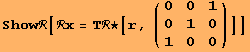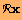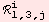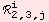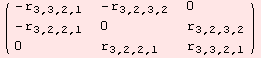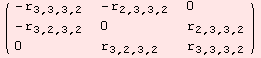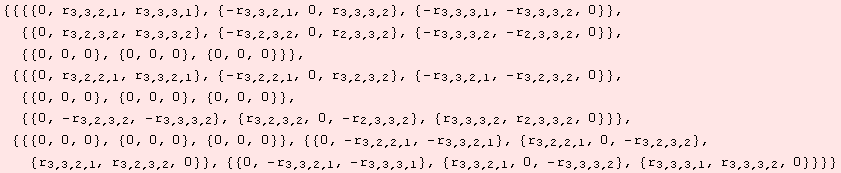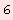Another useful constructor, produces a starting point for a commutator (1,2)-tensor of a Lie algebra.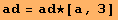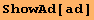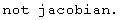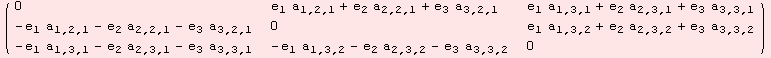However, as informed by the command ShowAd, the tensor does not (yet) satisfy the Jacobi identity. The Jacobi identity reduces to non-linear equations, so the set of all valid Lie algebra commutator tensors is not a vector space.

 Created by Mathematica  (September 30, 2006)